.The spreadsheets contain a very simple macro name f(x, y) that you can change for your own particular ODE. To change the function inside:

1. You use MS excel menu Tools - Macro - Visual Basic Editor or Press Alt-F11 together.
2. Go to Module 1 and change the function inside. For example you can type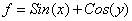to represent ODE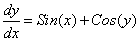or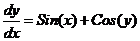or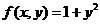to represent ODE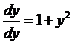(notice that in the last example, the independent variable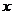does not exist in the right hand side of the ODE).
3. Save the change and go back to the Spreadsheet.
4. Change the initial value and the value of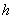to see the effect on the graph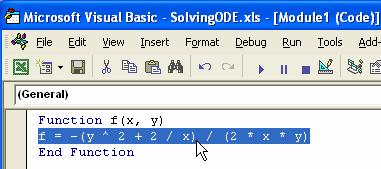References :

Riley, K. F., Hobson, M. P., and Bence, S. J. (2003) Mathematical Methods for Physics and Engineering, 2 ed., Cambridge University Press

Gernshenfeld, N. (1999) The Nature of Mathematical Modeling, Cambridge University Press

Chapra, S. C. and Canale, R. P. (1998) Numerical Methods for Engineers with Programming and Software Applications, 3 rd Ed., McGrawHill.

Preferable reference for this tutorial is

Teknomo, Kardi (2015) Solving Ordinary Differential Equation (ODE). https:\\people.revoledu.com\kardi\tutorial\ODE\